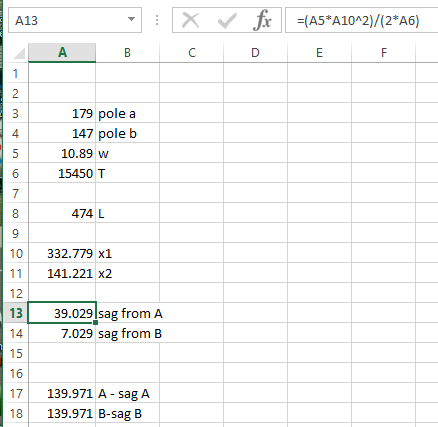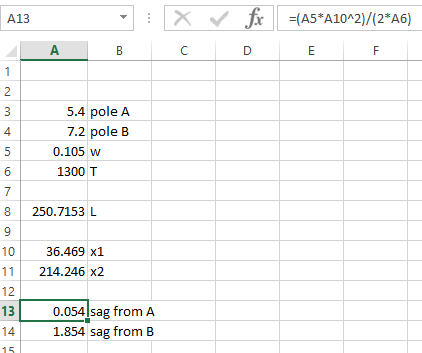Jump to content# Catenary function

## Recommended PostsGood day all!

I'm currently trying to create a function that will check if the user is drawing poles too far from each other. I was very easy to hard code, but I'm looking to make my code more dynamic.

I'd like to pass these variables on to the function:

Height Pole A

Height Pole B

Distance between pole A and B

Cable tension

Cable weight per unit length

Gravity (set as a constant)

All I need is to calculate the lowest point on the cable. I'm having a hard time translating formulas to LISP

We can only allow a sag of 1% compared to the pole height on the cable.

#### Share this post

##### Share on other sitesPlease post the code you have so far and the reference equations you are using for calculating the sag.  The equations at this site seem to be straightforward although LISP make make them ugly. Start by calculating x1 and x2 then go on to sag.  How do you determine the 1% sag limit with two poles each of different heights and potentially mounted at different elevations?

•1
•1

#### Share this post

##### Share on other sitesWhat @lrm said#### Share this post

##### Share on other sitesWHM your turn now if having problems with formula post what you are using rather than some one post a solution then you learn.

•1

#### Share this post

##### Share on other sitesSorry for the late response! I was away for Valentines.

So this is what I'm currently doing:

```(defun maxsag ( PH / sag)
(cond
((= "9" PH)(setq sag 0.9))
((= "7.2" PH)(setq sag 0.72))
((= "5.4" PH)(setq sag 0.54))
)
(princ sag)
)```

It's all hard coded, so when I hand it a new pole size, it won't know what to do, unless I hard code the new size in. Which is a horrible way to handle it.

Thanks for the link @lrm it is the clearest explanation I've seen. The 1% is 1% of the total pole height. I'll try my best to translate it into lisp.

Thanks for the help so far guys!

#### Share this post

##### Share on other sitesAlright so I'm one step closer!

Have a look at this so far!

```;w = weight per unit length
;l = span length
;h =  tension in on cable
(defun _maxsag ( w l h / l TL BL sag)
(setq l (* l l))
(setq TL (* w l));top line calculation
(setq BL (* 8 h));bottom line calculation
(setq sag (/ TL BL))
(princ sag)
)```

I now need to try calculating the max length if I know what the sag is, I'll update it soon.

#### Share this post

##### Share on other sitesAlright I think I've got it!

Man I haven't done this kind of math since high school!

Here I have two usable functions

```;Simple catenary function written by Wian Meier, with the guidance from people on cadtutor.

;w = weight per unit length
;l = span length
;h = tension in on cable
;s = The sag on the cable span

(defun _maxsag ( w l h / sag)
(setq sag (/ (* w (* l l)) (* 8 h)))
(princ sag)
)

(defun _maxlength ( w s h / l)
(setq l (sqrt (/ (* s (* 8 h)) w)))
(princ l)
)```

Let me know what you guys think!

Thanks for the help#### Share this post

##### Share on other sites@WHM in your original post implied that the two poles were of different height.  The equations you have in your code are for poles of the same height.  Look further down the page of the link I provided for the sag equation for unequal pole heights.

A minor note,   your statement:

`(setq sag (/ (* w (* l l)) (* 8 h)))`

could also be written

`(setq sag (/ (* w l l ) (* 8. h)))`

or

`(setq sag (/ (* w (expt l 2) ) (* 8. h)))`

Note, it is good practice to include the decimal point with the "8".   This ensure that real number math and not integer math will be done if h is an integer.  It might not be a problem here but could be in other equations when you divide two numbers and both are integers.

#### Share this post

##### Share on other sitesYou are right there are cases where I'd have different height poles, I'll sit tomorrow and work through the formula completely!

I'll post it once I get it or if I get stuck.

These functions are pretty handy! This enables me to automatically place poles on my utility cables, based on the cables characteristics.

#### Share this post

##### Share on other sitesPlease note that the equations that are stated at the website assume the cable takes the shape of a parabola when the true shape is a catenary (a.k.a. cycloid curve).  Several references state that if the sag is relatively small in comparison to the span (say the sag is less than about 10 percent of the span), then the catenary curve is very closely approximated by a parabolic curve (Source https://www.physicsforums.com/threads/wire-sag-formula.220848/).  A cable with the only load being its weight will take the shape of a catenary whereas a cable supporting a uniform horizontal load as with a suspension bridge will have a parabolic shape.  It sounds like for your application a parabolic assumption may be acceptable.

#### Share this post

##### Share on other sitesAm I understanding this correctly?

To calculate the sag on this scenario:

Pole A = 179

Pole B = 147

Weight per unit = 10.89

Tension = 15450

If I run this command:

`(_maxsag 10.89 (_straightlen (_HeigthDiff 179 147) 474) 15450)`

on this code:

```;w = weight per unit length
;l = span length
;h = tension in on cable
;s = The sag on the cable span

(defun _maxsag ( w l h / sag)
(setq sag (/ (* w l l) (* 8. h)))
)

(defun _maxlength ( w s h / l)
(setq l (sqrt (/ (* s (* 8. h)) w)))
)

;h1 = Height on support 1
;h2 = Height on support 2
;h  = difference in height

(defun _HeigthDiff ( h1 h2 / h)
(setq h (abs (- h1 h2)))
)

;sl = Straightline distance between two points
;h  = difference in height
;s  = horizontal distance between two points

(defun _straightlen ( h s / s1 )
(setq sl (sqrt (+ (* h h) (* s s))))
)```

I get 19.8857m sag.

So to my understanding the _straightlen function in combination with _HeightDiff essentially rotates the cable line so that Pole A and Pole B is at the same level.

From there I can use the _maxsag function to determine what the sag is.

Am I correct in assuming that 19.8857 will be my sag with two different size poles?

Thanks for all the help so far!

#### Share this post

##### Share on other sitesI created an Excel spreadsheet using the equations in the referenced link and the values from you last post.  x1 and x2 are first determined and then sag1 (from A) and sag2 (from B).  As a check I subtracted the sag from A and compared it to the sag from B.  The bottom point of the cable has an elevation of 139.971m.Since your input consists of 5 values why not make one function that accepts or prompts for the 5 numbers and then computes x1, x2 sag1 and sag2,  You really only need x1 and sag1 to give you the result.  The other values are just a check.

Your program would look something like:

```(defun c:maxsag (    /    )
(setq polea (getreal "\nEnter pole A height.")
poleb (getreal "\nEnter pole B height.")
... continue for the other input values
...

(setq x1 (...
sag1 (...
(setq sagele  (- polea sag1))
(princ "\nElevation of lowest point of sagging cable is: ")
(princ sagele)
...

)```

If you would prefer to reference geometry in your drawing you could modify the input to use getpoint instead of getreal.

#### Share this post

##### Share on other sitesThanks for this, I'm learning quite a lot!

The main thing I'm trying to make with this, is add this to a whole framework I'm writing. Poles get placed from the top down, so you'll never see the cable curve. All pole and cable characteristics gets saved within their own dictionaries & xrecords, so the info will be pulled from dictionaries.

I'm trying to prevent my draughtsman from accidentally placing a pole to far away from the previous one. The sag will always be set to 1% of the poles length. If you look at the (_maxlength) function I've written it does exactly that, but it assumes that poles are all the same height, where we do have rare occasions where we'll have a longer pole.

Here is more realistic cable specs:

Pole A = 5.4 (so max sag will be 0.054)

Pole B = 7.2 (so max sag will be 0.072)

Weight per unit = 0.105

Tension = 1300

This has been a great learning curve (pun intended) so far!

#### Share this post

##### Share on other sitesTo better understand your objective I set up the simple Excel worksheet I referenced in an earlier post.  I assume the goal for the max sag should be applied to the shorter of the two poles as this point will obviously be lower than the sag for the taller pole otherwise there might be zero sag for the shorter pole.  Is that correct?

Using Excel's Goal Seek function and setting a goal of 0.054 for the sag at A it calculates a value for L of 250.7.  Does that seem reasonable?## Join the conversation

You can post now and register later. If you have an account, sign in now to post with your account.
Note: Your post will require moderator approval before it will be visible.Reply to this topic...

×   Pasted as rich text.   Paste as plain text instead

Only 75 emoji are allowed.

×   Your link has been automatically embedded.   Display as a link instead

×   Your previous content has been restored.   Clear editor

×   You cannot paste images directly. Upload or insert images from URL.

×

×
×
• Create New...